Courses

# Einsteins A and B Coefficients and Expression for Energy Density Civil Engineering (CE) Notes | EduRev

## Civil Engineering (CE) : Einsteins A and B Coefficients and Expression for Energy Density Civil Engineering (CE) Notes | EduRev

The document Einsteins A and B Coefficients and Expression for Energy Density Civil Engineering (CE) Notes | EduRev is a part of the Civil Engineering (CE) Course Engineering Physics - Notes, Videos, MCQs & PPTs.
All you need of Civil Engineering (CE) at this link: Civil Engineering (CE)

Einsteins A and B Coefficients and Expression for energy density
Let E1 and E2 be the two energy states of the system of atoms. Let N1 and N2 be the number of atoms with energy states E1 and E2 respectively. Let Uν represent the energy density of incident photon having frequency (ν). Hence the system absorbs and emits energy through the following processes

(a)Stimulated Absorption:
In case of Stimulated absorption, the rate of absorption is given by number of absorptions per second per unit volume. Rate of absorption depends upon, (1) Number of atoms in the lower energy state. (N1)
(2) The energy density (Uν).
Hence, the Rate of absorption ∝ N1Uν and Rate of absorption = B12N1Uν, where B12 is proportionality constant called Einsteins coefficient of induced absorption.

(b)Spontaneous emission:
In case of Spontaneous emission, the rate of spontaneous emission is given by number of spontaneous emissions per second per unit volume. Rate of Spontaneous emission depends upon,
(1) Number of atoms in the higher energy state (E2). i.e. (N2)
Hence, the Rate of Spontaneous emission ∝ N2
Rate of Spontaneous emission = A21N2.
Where A21 is proportionality constant Called Einsteins coefficient of Spontaneous emission.

(c)Stimulated Emission:
In case of Stimulated Emission The rate of Stimulated emission is given by number of Stimulated emissions per second per unit volume. Rate of Stimulated Emission depends upon,
(1) Number of atoms in the higher energy state (E2). i.e. (N2)
(2) The energy density (Uν)
Hence, the Rate of Stimulated emission ∝ N2
Rate of Stimulated emission = B21 N2 Uν, where B21 is proportionality constant Called Einsteins coefficient of Stimulated Emission.

Under Thermal Equilibrium the total Energy of the System remains unchanged. Hence number of photons absorbed is equal to number of photons emitted. Rate of Induced Absorption = Rate of Spontaneous emission + Rate of Stimulated Emission. Therefore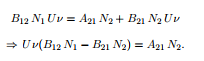After simplification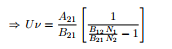But according to the Boltzman’s law of distribution the number of atoms in the higher energy state E2 at any temperature “T” is given by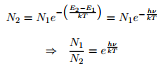Substituting for N1/N2 in equation (5.1)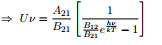According to Planck’s Law of Radiation. The equation for Energy density is given by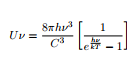Comparing (5.2) and (5.3) we have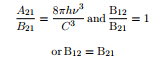(i.e. Probability of Stimulated absorption is equal to Probability of Stimulated emission.) Hence A21 and B21 can be replaced by A and B and the expression for the Energy density is given by,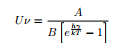Where h is Plancks constant, k is Boltzmann Constant, T is Absolute Temperature, and c is the velocity of light.

Offer running on EduRev: Apply code STAYHOME200 to get INR 200 off on our premium plan EduRev Infinity!

,

,

,

,

,

,

,

,

,

,

,

,

,

,

,

,

,

,

,

,

,

;